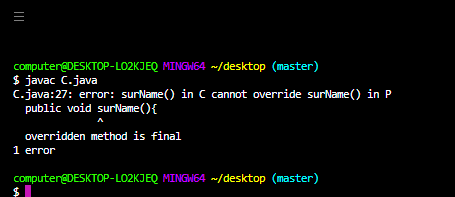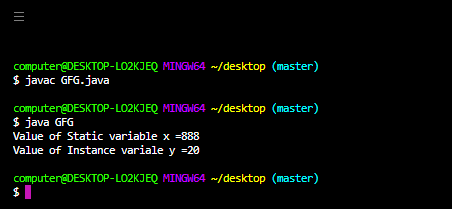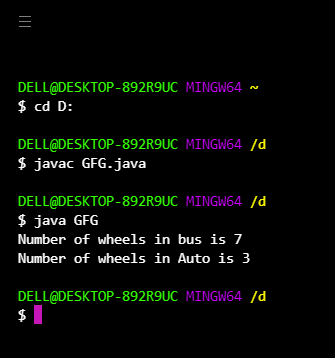Open in App
Not now

# Final vs Static vs Abstract Non-Access Modifier

• Last Updated : 04 Mar, 2022

Modifiers are specific keywords present in Java using that we can make changes to the characteristics of a variable, method, or class and limit its scope. Java programming language has a rich set of Modifiers. Modifiers in Java are divided into two types

1. Access Modifiers
2. Non-Access modifiers

Non-access modifiers provide information about the characteristics of a class, method, or variable to the JVM. Seven types of Non-Access modifiers are present in Java. They are

• static
• final
• abstract
• synchronized
• volatile
• transient
• native

Read more about Non-Access Modifiers in Java. In this article, we are going to discuss the differences among Final, Static, and Abstract Non-Access Modifiers.

### Final Non-Access Modifier

The final non-access modifier is applicable to classes, methods, and variables. If we declare a parent class method as final then we can’t override that method in the child class because its implementation is final and if a class is declared as final we can’t extend the functionality of that class i.e we can’t create a child class for that class i.e inheritance is not possible for final classes. Every method present inside the final class is always final y default but every variable present inside the final class need not be final. The main advantage of the final keyword is we can achieve security and we can provide a unique implementation. But the main disadvantage of the final keyword is we are missing key benefits of OOPs like Inheritance(Because of the final class), Polymorphism(Because of the final method)  hence if there are no specific requirements then it is not recommended to use the final keyword.

Example 1:

## Java

 `// Java Program to illustrate Final keyword` `// Where No final keyword Is Used`   `// Importing required classes` `import` `java.io.*;` `import` `java.util.*;`   `// Class 1` `// Super-class` `class` `P {`   `    ``// Method 1` `    ``// To declare first name` `    ``public` `void` `firstName()` `    ``{`   `        ``// Passing name and print it` `        ``System.out.println(``"Mayank"``);` `    ``}`   `    ``// Method 2` `    ``// To declare last name` `    ``public` `void` `surName()` `    ``{`   `        ``// Passing name and print it` `        ``System.out.println(``"Trivedi"``);` `    ``}` `}`   `// Class 2` `// Sub-class` `// Extending above class` `class` `C ``extends` `P {`   `    ``// Method 1` `    ``// Trying to override the last name` `    ``public` `void` `surName()` `    ``{` `        ``// Display surname` `        ``System.out.println(``"Sharma"``);` `    ``}`   `    ``// Method 2` `    ``// Main driver method` `    ``public` `static` `void` `main(String[] args)` `    ``{`   `        ``// Display message` `        ``System.out.println(``"GFG"``);` `    ``}` `}`

Output:

GFG

Example 2:

## Java

 `// Java Program to illustrate Final keyword` `// When final keyword Is Used`   `// Importing required classes` `import` `java.io.*;` `import` `java.util.*;`   `// Class 1` `// Super-class` `class` `P {`   `    ``// Method 1` `    ``// To declare first name` `    ``public` `void` `firstName()` `    ``{`   `        ``// Passing name and print it` `        ``System.out.println(``"Mayank"``);` `    ``}` `      `  `      ``// Method 2` `    ``// To declare last name` `    ``public` `final` `void` `surName()` `    ``{`   `        ``// Passing name and print it` `        ``System.out.println(``"Trivedi"``);` `    ``}` `}`   `// Class 2` `// Sub-class` `// Extending above class` `class` `C ``extends` `P {`   `    ``// Method 1` `    ``// Trying to override the last name` `    ``public` `void` `surName()` `    ``{` `        ``// Display surname` `        ``System.out.println(``"Sharma"``);` `    ``}` `    `  `      ``// Method 2` `    ``// Main driver method` `    ``public` `static` `void` `main(String[] args)` `    ``{`   `        ``// Display message` `        ``System.out.println(``"GFG"``);` `    ``}` `}`

Output:### Static Non-Access Modifier

The static non-access modifier is applicable for methods and variables but not for classes. We can declare a top-level class with a static modifier but we can declare the inner class as static (such types of inner classes are known as static nested classes). In the case of instance variable for every object, a separate copy will be created but in the case of static variable, a single copy will be created at class level and shared by every object of that class.

Example:

## Java

 `// Java Program to Illustrate Static Access Modifier`   `// Importing required classes` `import` `java.io.*;` `import` `java.util.*;`   `// Main class` `class` `GFG {`   `    ``// Creating a static variable and` `    ``// initializing a custom value` `    ``static` `int` `x = ``10``;`   `    ``// Creating a instance variable and` `    ``// initializing a custom value` `    ``int` `y = ``20``;`   `    ``// Main driver method` `    ``public` `static` `void` `main(String[] args)` `    ``{`   `        ``// Creating an object of class inside main() method` `        ``GFG t1 = ``new` `GFG();`   `        ``// Accessing and re-initializing the` `        ``// static and instance variable` `        ``// using t1 reference` `        ``t1.x = ``888``;` `        ``t1.y = ``99``;`   `        ``// Creating an object of class inside main() method` `        ``// again` `        ``GFG t2 = ``new` `GFG();`   `        ``// Accessing the static and instance variable using` `        ``// t2 reference as we know that for each object` `        ``// there is separate copy of instance variable` `        ``// created. While same copy of static variable will` `        ``// be shared between the objects`   `        ``// Displaying the value of static and instance` `        ``// variable using t2 object reference` `        ``System.out.println(` `            ``"Value of Static variable x = "` `+ t2.x + ``"\n"` `            ``+ ``"Value of Instance variable y = "` `+ t2.y);` `    ``}` `}`

Output:### Abstract Non-Access Modifier

The abstract non-access modifier is applicable only for classes and methods but not for variables. If we declare any method as abstract then that method must have its implementation in the child class of the respective class because abstract methods never talk about implementation. If any modifier talks about implementation then it forms an illegal combination with an abstract modifier. In a similar way if for any java class if we are not allowed to create an object (because of partial implementation) then such type of class we have to declare with abstract modifier.

Example:

## Java

 `// Java program to illustrate Abstract Access Modifier` ` `  `// Importing the required packages` `import` `java.io.*;` `import` `java.util.*;` ` `  `// Class 1` `// Helper abstract class` `abstract` `class` `Vehicle {` ` `  `    ``// Declaring an abstract method getNumberOfWheel` `    ``abstract` `public` `int` `getNumberOfWheel();` `}` ` `  `// Class 2` `// Helper class extending above abstract class` `class` `Bus ``extends` `Vehicle {` ` `  `    ``// Giving the implementation` `    ``// to the  parent abstract method` `    ``public` `int` `getNumberOfWheel() { ``return` `7``; }` `}` ` `  `// Class 3` `// Helper class extending above abstract class` `class` `Auto ``extends` `Vehicle {` ` `  `    ``// Giving the implementation` `    ``// to the  parent abstract method` `    ``public` `int` `getNumberOfWheel() { ``return` `3``; }` `}` ` `  `// Class 4` `// Main class` `public` `class` `GFG {` ` `  `    ``// Main driver method` `    ``public` `static` `void` `main(String[] args)` `    ``{` ` `  `        ``// Creating Bus object` `        ``Bus b = ``new` `Bus();` ` `  `        ``// Creating Auto object` `        ``Auto a = ``new` `Auto();` ` `  `        ``// Now getting and displaying` `        ``// the number of wheels` `        ``// for Bus by calling the` `        ``// getNumberOfWheel method` `        ``System.out.println(``"Number of wheels in bus is"` `                           ``+ ``" "` `+ b.getNumberOfWheel());` ` `  `        ``// Now getting and displaying` `        ``// the number of wheels` `        ``// for Auto by calling the` `        ``// getNumberOfWheel method` `        ``System.out.println(``"Number of wheels in Auto is"` `                           ``+ ``" "` `+ a.getNumberOfWheel());` `    ``}` `}`

Output:### Difference Table

My Personal Notes arrow_drop_up
Related Articles